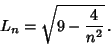## Markov Number

The Markov numbersoccur in solutions to the Diophantine Equationand are related to Lagrange NumbersbyThe first few solutions are, (1, 1, 2), (1, 2, 5), (1, 5, 13), (2, 5, 29), .... The solutions can be arranged in an infinite tree with two smaller branches on each trunk. It is not known if two different regions can have the same label. Strangely, the regions adjacent to 1 have alternate Fibonacci Numbers 1, 2, 5, 13, 34, ..., and the regions adjacent to 2 have alternate Pell Numbers 1, 5, 29, 169, 985, ....

Letbe the number of Triples with, thenwhere(Guy 1994, p. 166).

See also Hurwitz Equation, Hurwitz's Irrational Number Theorem, Lagrange Number (Rational Approximation) Liouville's Rational Approximation Theorem, Liouville-Roth Constant, Roth's Theorem, Segre's Theorem, Thue-Siegel-Roth Theorem

References

Conway, J. H. and Guy, R. K. The Book of Numbers. New York: Springer-Verlag, pp. 187-189, 1996.

Guy, R. K. Markoff Numbers.'' §D12 in Unsolved Problems in Number Theory, 2nd ed. New York: Springer-Verlag, pp. 166-168, 1994.